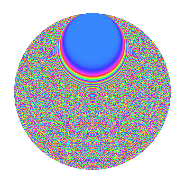# Properties

 Label 525.4.bsLevel $525$ Weight $4$ Character orbit 525.bs Rep. character $\chi_{525}(2,\cdot)$ Character field $\Q(\zeta_{60})$ Dimension $3776$ Sturm bound $320$

# Related objects

## Defining parameters

 Level: $$N$$ $$=$$ $$525 = 3 \cdot 5^{2} \cdot 7$$ Weight: $$k$$ $$=$$ $$4$$ Character orbit: $$[\chi]$$ $$=$$ 525.bs (of order $$60$$ and degree $$16$$) Character conductor: $$\operatorname{cond}(\chi)$$ $$=$$ $$525$$ Character field: $$\Q(\zeta_{60})$$ Sturm bound: $$320$$

## Dimensions

The following table gives the dimensions of various subspaces of $$M_{4}(525, [\chi])$$.

Total New Old
Modular forms 3904 3904 0
Cusp forms 3776 3776 0
Eisenstein series 128 128 0

## Trace form

 $$3776q - 8q^{3} - 20q^{4} - 24q^{6} - 44q^{7} - 10q^{9} + O(q^{10})$$ $$3776q - 8q^{3} - 20q^{4} - 24q^{6} - 44q^{7} - 10q^{9} + 8q^{10} + 84q^{12} - 64q^{13} + 136q^{15} - 7180q^{16} - 24q^{18} - 20q^{19} - 12q^{21} - 1488q^{22} + 56q^{25} - 8q^{27} - 96q^{28} + 490q^{30} - 12q^{31} + 420q^{33} - 80q^{34} + 488q^{36} - 232q^{37} - 2450q^{39} + 1276q^{40} + 890q^{42} + 1280q^{43} - 2820q^{45} - 12q^{46} - 1484q^{48} - 16q^{51} - 408q^{52} - 10q^{54} - 1320q^{55} - 4708q^{57} + 1552q^{58} - 5640q^{60} - 12q^{61} + 5854q^{63} - 80q^{64} - 870q^{66} + 992q^{67} - 6200q^{69} + 2360q^{70} - 876q^{72} - 2824q^{73} + 1824q^{75} + 896q^{76} - 7468q^{78} - 20q^{79} - 6q^{81} + 2532q^{82} + 1900q^{84} - 4816q^{85} + 42q^{87} - 5264q^{88} + 25604q^{90} - 24q^{91} - 3044q^{93} - 20q^{94} + 90q^{96} + 18464q^{97} + O(q^{100})$$

## Decomposition of $$S_{4}^{\mathrm{new}}(525, [\chi])$$ into newform subspaces

The newforms in this space have not yet been added to the LMFDB.

## Hecke characteristic polynomials

There are no characteristic polynomials of Hecke operators in the database